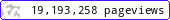•••••MadAsMaths.com :: Maths Booklets :: Standard Topics :: Various

STANDARD TOPICS - VARIOUS

These booklets are suitable for

• the second year material, except integration and trigonometry, of a two year course in A Level mathematics.

• revision/introduction to various mathematical topics, except integration and trigonometry, for undergraduate students in various science degrees or diplomas.

 binomial_series_expansions_exam_questions.pdfbinomial_series_expansions_practice.pdfcurve_sketching_exam_questions.pdfdifferentiation_ii_exam_questions.pdfdifferentiation_practice_ii.pdfdifferentiation_practice_ii_student_version.pdfdifferentiation_practice_ii_exam_questions.pdfequations_advanced_skills.pdfexponentials_logarithms_exam_questions.pdfexponentials_logarithms_practice.pdffunction_exam_questions.pdffunction_practice.pdfimplicit_differentiation.pdfimplicit_equations_exam_questions.pdfinequalities.pdfmodulus_function_exam_questions.pdfmodulus_function_practice.pdfnumerical_solutions_of_equations.pdfparametric_equations.pdfparametric_equations_exam_questions.pdfpolynomials.pdfrelated_rates_of_change.pdftransformations_of_graphs_exam_questions.pdftransformations_of_graphs_practice.pdftransformations_of_graphs_practice_student_version.pdf